# HC Verma Solutions Class 12 Chapter 13 Magnetic Field Due to Current

HC Verma Solutions for Class 12 Chapter 13 Magnetic Field Due to Current is intended for students who want to learn the topics clearly and know the right answers to the questions and exercises given in the HC Verma book. In this chapter, students will deal with the topic of magnetic flux density and magnetic field strength. Students will also learn about some SI units and solve some problems.

The HC Verma solutions provided here will help students develop a proper approach and equip them with foundational knowledge that will be helpful while tackling questions in the exams. Additionally, students will get deeper insights into complex topics and they will also develop better numerical solving skills. Students who want to excel in their academics and exams like IIT JEE can download and use the solutions.

## Key Topics Related To Magnetic Field Due to Current

Some of the key concepts discussed in this chapter include;

• Biot-Savart Law
• Magnetic Field Due to Current in a Straight Wire
• Force Between Parallel Currents
• Field due to Circular Current
• Ampere’s Law
• Magnetic Field at a Point due to Long Straight Current
• Solenoid
• Toroid

## Class 12 Important Questions In Chapter 13

1. We are looking at a circular wire carrying an electric current. The flow of the current is clockwise. In which way will the field at the centre move? Come towards you or go away from you?

2. While using electrical appliances, the connecting wires carrying currents in opposite directions are usually twisted together. Explain, the purpose of this setup and how it avoids unwanted magnetic fields?

3. A proton beam travels from north to south and an electron beam moves from south to north. If the electron beam is not affected by the earth’s magnetic field in which direction will the beam be deflected?

(a) upwards (b) away from the proton beam (c) downwards (d) towards the proton beam

4. When a charged particle is moved along a magnetic field line where will the magnetic force on the particle be present at?

(a) perpendicular to its velocity (b) along its velocity (c) opposite to its velocity (d) zero.

5. Which of the following will a moving charge produce?

(a) A magnetic field (b) An electric field (c) Both (d) None of them

## HC Verma Solutions Vol 2 Magnetic Field Due to Current Chapter 13

Question 1: Using the formulae F = qv x B and B = μ0i/2πr show that the S.I. units of the magnetic field B and the permeability constant μ0 may be written as N m–1A–1 2nd NA–1 respectively.

Solution: Using given formulas,

Magnetic field = B = F/qv = F/itv

[we know, Charge: q = it]

Now,

Units of Force (F) = N

Units of Current (i) = A

Units of time (t) = s

Units of velocity (v) = m/s

Unit of Magnetic field : B = N m-1A-1.

Again, using relation, B = μ0i/2πr

or μ0 = B2πr/i = N m-1A-1 x m/A = NA-2

Hence proved

Question 2: A current 10A is established in a long wire along the positive z-axis. Find the magnetic filed B at the point (1m, 0, 0).

Solution:

Ampere’s Law for a current carrying straight wire: B = μ0i/2πd

μ0 = 4π × 10-7 T mA-1

and d = distance between the current carrying wire and the required point.

=> B = [4π × 10-7 x 10]/[2πx1]

=> B = 2 x 10-6 T

Question 3: A copper wire of diameter 1.6 mm carries a current of 20 A. Find the maximum magnitude of the magnetic field due to this current.

Solution:

Diameter of the copper wire: d = 1.6mm = 1.6× 10-3 m

Current through the wire: I = 20 A

Ampere’s Law for a current carrying wire of cross section area: B = μ0i/2πr

μ0 = 4π × 10-7 T mA-1 and Radius of wire : r = d/2 = 8× 10-3 m

B = [4π × 10-7 x 20]/[ 2π × 8×10-3]

=> B = 5 x 10-3 T

Question 4: A transmission wire carries a current of 100 A. What would be the magnetic field B at a point on the road if the wire is 8 m above the road?

Solution:

Diameter of the copper wire: d = 8 m

Current through the transmission wire: I = 100 A

Ampere’s Law for a current carrying wire of cross section area: B = μ0i/2πd

μ0 = 4π × 10-7 T mA-1

Substituting values, we get

B = [4π × 10-7 x 100]/[ 2π × 8]

=> B = 2.5 x 10-6 T

Question 5: A long, straight wire carrying a current of 1.0 A is placed horizontally in a uniform magnetic field B = 1.0 × 10–8 T pointing vertically upward figure. Find the magnitude of the resultant magnetic field at the points P and Q, both situated at a distance of 2.0 cm from the wire in the same horizontal plane.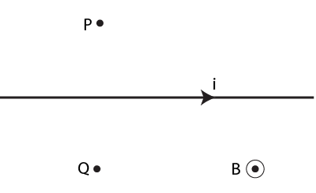Solution:

Magnitude of Horizontal magnetic field: Bo = 1.0 × 10–5 T

Current in the wire = i = 1.0 A

Distance between points P, Q and the wire = d = 2.0 cm = 0.02 m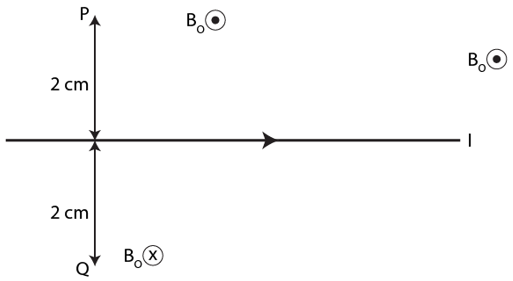Cross determines the field going into the plane and dot determines the field coming out of the field.

Bw is the magnetic field due to wire:

Ampere’s Law for a current carrying wire: B = μ0i/2πd

μ0 = 4π × 10-7 T mA-1

Bw = [4π × 10-7 x 1]/[ 2π × 0.02]

=> Bw = 1 x 10-5 T

Resultant magnetic field at point P,

BP = Bo +Bw

BP = 1× 10-5 + 1× 10-5 = 2 × 10-5 T

Magnetic field at point Q due to wire,

Bw = – [4π × 10-7 x 1]/[ 2π × 0.02]

=> Bw = – 1 x 10-5 T

The -ve sign is due to the field at Q is opposite to the direction of horizontal field and at P.

Resultant magnetic field at point Q,

BQ = Bo +Bw

BQ = 1× 10-5 – 1× 10-5 = 0

Question 6: A long, straight wire of radius r carries a current i and is placed horizontally in a uniform magnetic field B pointing vertically upward. The current is uniformly distributed over its cross section.

(a) At what points will the resultant magnetic field have maximum magnitude?

(b) What will be the minimum magnitude of the resultant magnetic field?

Solution: Wire carrying current generate a magnetic field around it. If wire is straight then it will be maximum at the mid point.

Magnetic field generated by wire = μ0i/2πr

net magnetic field = B + μ0i/2πr

(b) B= 0 when r < μ0i/2πB

Also, B = 0 when r = μ0i/2πB

But when r > μ0i/2πB

Net magnetic field = B – μ0i/2πr

Question 7: A long, straight wire carrying a current of 30 A is placed in an external, uniform magnetic field of 4.0 ×10-4 T parallel to the current. Find the magnitude of the resultant magnetic field at a point 2.0 cm away from the wire.

Solution:

Current in the wire = I = 30 A

External Uniform magnetic field = BO = 4.0 ×10–4 T

Distance between the point and wire = d = 2 cm = 0.02 m

Here B is the magnetic field due to wire. BO is parallel to the current in the wire.

And, BO is perpendicular to B.

Magnetic field due to current in wire = B = μ0i/2πd

= [2×10-7x30]/0.02

= 3 x 10-4 T

So, resultant magnetic field:

Bnet2 = B2 + B02

Bnet2 = (4 x 10-4)2 + (3 x 10-4)2

Or Bnet = 5 x 10-4 T

Question 8: A long, vertical wire carrying a current of 10 A in the upward direction is placed in a region where a horizontal magnetic field of magnitude 2.0 × 10-3 T exists from south to north. Find the point where the resultant magnetic field is zero.

Solution:

For the resultant magnetic field to be zero, the magnetic field due to the wire should be of the same magnitude as BO and in the direction north to south.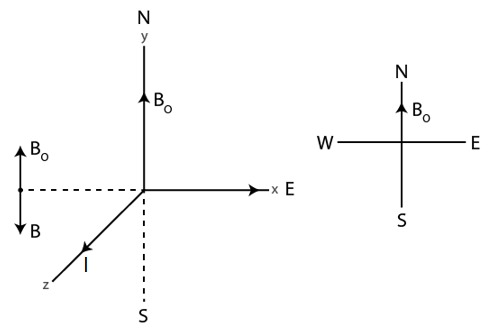Magnetic field due to current in wire = B = μ0i/2πd

We have B = Bo to get zero resultant.

=> 2 x 10-3 = μ0i/2πd

=> d = [4πx10-7 x 10]/[ 2π x 2 x 10-3]

=> d = 0. 001 m = 1 mm

Point should be place at a distance of 0.001 m from the wire in west direction

Question 9: Figure shows two parallel wires separated by a distance of 4.0 cm and carrying equal currents of 10 A along opposite directions. Find the magnitude of the magnetic field B at the points A1, A2, A3 and A4.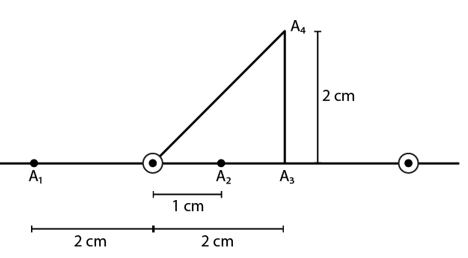Solution: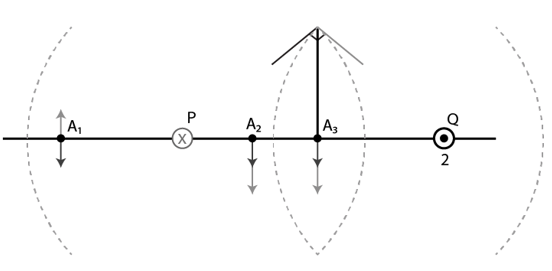P and Q are the two wires having opposite direction of current.

P has current in the plane and Q has current that comes from the plane. According to the right rule, P has a field that rises at A1 and falls at A2 and A3 and is tangent to A4, as shown by the red arrow.

Similarly, Q will have a field going down as A1, A2 and A3 and touching A4 as shown by the blue arrow.

Magnetic field due to current in wire = B = μ0i/2πd [Formula used]

At A1 :

Net magnetic field due to P and Q

Bnet = BP – BQ

= μ0i/2πd1 – μ0i/2πd2

Given:

PA1 = d1 = 0.02 m

QA1 = d2 = 0.06m

=> Bnet = μ0i/2π [1/0.02 – 1/0.06]

=> Bnet = [(4 πx 10-7 x 10)/2 π] x 33.33 = 6.66 x 10-5 T

At A2 :

Net magnetic field due to P and Q

Bnet = BP + BQ

= μ0i/2πd1 + μ0i/2πd2

Given:

PA2 = d1 = 0.01 m

QA3 = d2 = 0.03 m

=> Bnet = μ0i/2π [1/0.01 + 1/0.03]

=> Bnet = [(4 πx 10-7 x 10)/2 π] x 133.33 = 2.66 x 10-4 T

At A3 :

Net magnetic field due to P and Q

Bnet = BP + BQ

= μ0i/2πd1 + μ0i/2πd2

Given:

PA3 = d1 = 0.02 m

QA3 = d2 = 0.02 m

=> Bnet = μ0i/2π [1/0.02 + 1/0.02]

=> Bnet = [(4 πx 10-7 x 10)/2 π] x 100 = 2.0 x 10-4 T

At A4 :

Net magnetic field due to P and Q

Bnet2 = BP2 + BQ2 …(1)

[By Pythagoras theorem]

= μ0i/2πd1 + μ0i/2πd2

(PA4)2 = (PA3)2 + (A3A4)2 = 0.022 + 0.022

=> (PA4) = 0.028 m = d1

Now, BP = μ0i/2πd1 = [(4 πx 10-7 x 10)/(2 πx0.028] = 7.14 x 10-5 T

Similarly, QA4 = 0.028 m = d2

So, BQ = μ0i/2πd2 = [(4 πx 10-7 x 10)/(2 πx0.028] = 7.14 x 10-5 T

On substituting the values, (1)=>

Bnet = 1 x 10-4 T, which is the magnetic field at A4.

Question 10: Two parallel wires carry equal currents of 10 A along the same direction and are separated by a distance of 2.0 cm. Find the magnetic field at a point which is 2.0 cm away from each of these wires.

Solution:Above circles show the magnetic field in a plane generated due to wires carrying current.

Here triangle PI1I2 is an equilateral triangle.

=> Each angle of the triangle = 600.

The magnetic field at point P:

Bnet2 = B12 + B22 + 2 B1 B2 cos 60o ……(1)

Here B1 = B2 = [2×10-7x10]/[2×10-2] and cos60o = ½

On substituting the values and solving, we have

Bnet = √3 x 10-4 T = 1.732 x 10-4 T

Question 11: Two long, straight wires, each carrying a current of 5A, are placed along the x-and y-axis respectively. The currents point along the positive directions of the axes.

Find the magnetic fields at the points (a) (1m, –1m), (b) (–1m, 1m) (c) (–1m, –1m) and (d) (1m, –1m).

Solution:

The magnitude of magnetic field due to current in the wire = B1 = B2 = μ0i/2πd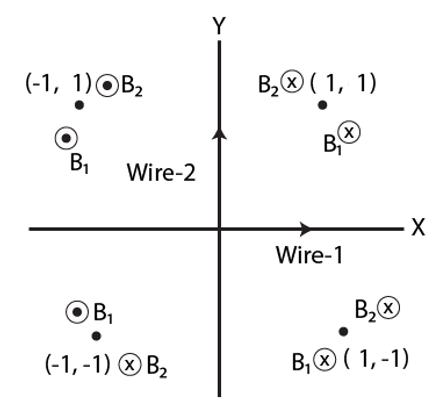(a) At point (1m, 1m)

The magnetic fields due to wire 1 and 2 are in opposite direction and same magnitude. Hence, net magnetic field is zero.

(b) At (-1m, 1m)

The magnetic field due to wire 1 and 2 add as direction of magnetic field is same.

Bnet = B1 + B2

= [2×10-7x5]/1 + [2×10-7x5]/1

= 2 x 10-6 T (along z-axis)

(c) At (-1m, -1m)

The magnetic field due to wire 1 and 2 are same in magnitude and opposite in direction.

Bnet = B1 + B2 = 0

(d) At (1m, 1m)

The magnetic field due to wire 1 and 2 are in upward direction.

Bnet = B1 + B2

= [2×10-7x5]/1 + [2×10-7x5]/1

= 2 x 10-6 T (along negative z-axis)

Question 12: Four long, straight wires, each carrying a current of 5.0 A, are placed in a plane as shown in figure. The points of intersection from a square of side 5.0 cm.

(a) Find the magnetic field at the centre P of the square

(b) Q1, Q2, Q3 and Q4 are points situated on the diagonal of the square and at a distance from P that to equal to the length of the diagonal of the square. Find the magnetic fields at these points.

Solution: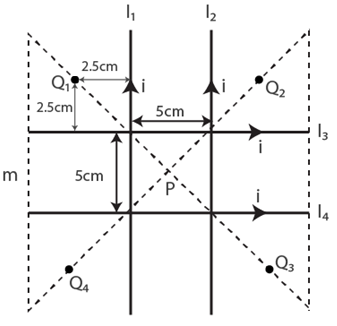(a) Consider point P.

Magnetic field due to wires, l1 and l2 are the same in magnitude but opposite in direction. In same way, magnetic field due to wires, l3 and l4 are the same in magnitude but opposite in direction.

Hence, net magnetic field is zero.

(b) Consider point Q1.

Distance between wire l1 and Q1 = d1 = 0.025 m

Distance between wire l2 and Q1 = d2 = 0.075 m

Distance between wire l3 and Q1 = d3 = 0.025 m

Distance between wire l4 and Q1 = d4 = 0.075 m

Net magnetic field at Q1 due to all 4 wires:

BQ1 = Bl1 + Bl2 + Bl3 + Bl4

= μ0i/2πd1 + μ0i/2πd2 + μ0i/2πd3 + μ0i/2πd4

= [4πx10-7x5]/2π [1/0.025 + 1/0.075 + 1/0.025 + 1/0.075]

= 1.06 x 10-4 T [upward direction]

Consider point Q2.

Distance between wire l1 and Q2 = d1 = 0.075 m

Distance between wire l2 and Q2 = d2 = 0.025 m

Distance between wire l3 and Q2 = d3 = 0.025 m

Distance between wire l4 and Q2 = d4 = 0.075 m

Net magnetic field at Q2 due to all 4 wires:

BQ2 = Bl1 + Bl2 + Bl3 + Bl4

= μ0i/2πd1 + μ0i/2πd2 – μ0i/2πd3 – μ0i/2πd4

= [4πx10-7x5]/2π [1/0.075 + 1/0.025 – 1/0.025 – 1/0.075]

= 0

Consider point Q3.

Distance between wire l1 and Q3 = d1 = 0.075 m

Distance between wire l2 and Q3 = d2 = 0.025 m

Distance between wire l3 and Q3 = d3 = 0.075 m

Distance between wire l4 and Q3 = d4 = 0.025 m

Net magnetic field at Q3 due to all 4 wires:

BQ3 = Bl1 + Bl2 + Bl3 + Bl4

= μ0i/2πd1 + μ0i/2πd2 + μ0i/2πd3 + μ0i/2πd4

= [4πx10-7x5]/2π [1/0.075 + 1/0.025 + 1/0.075 + 1/0.025]

= 1.06 x 10-4 T [downward direction]

At Q4

Magnetic field at Q1 and Q3 have same magnitude of 1.06 × 10-4 T but in opposite direction and magnetic field at Q2 and Q4 is zero.

Question 13: Figure shows a long wire bent at the middle to from a right angle. Show that the magnitudes of the magnetic fields at the points, P, Q, R and S are equal and find this magnitude.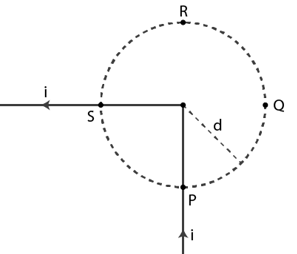Solution: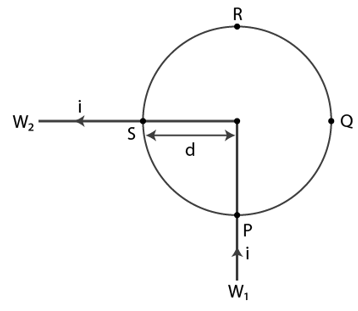At point P,

Magnetic field at P due to W1 is zero as P is on the axis of W1.

B1 = 0

Magnetic field at P due to W2:

B2 = μ0i/4πd

Which is perpendicular to the plane in outward direction.

So Bnet = B1 + B2 = μ0i/4πd [perpendicular to the plane in outward direction.]

At point Q:

Magnetic field due to W1:

B1 = μ0i/4πd [perpendicular to the plane in inward direction]

Magnetic field due to W2:

B2 = 0

=> Bnet = B1 + B2 = μ0i/4πd [perpendicular to the plane in inward direction]

At point R:

=> Bnet = μ0i/4πd [perpendicular to the plane in inward direction]

At point S:

Magnetic field due to W1:

B1 = μ0i/4πd [perpendicular to the plane in outward direction]

Magnetic field due to W2:

B2 = 0

=> Bnet = B1 + B2 = μ0i/4πd [perpendicular to the plane in outward direction]

Question 14: Consider a straight piece of length x of a wire carrying a current i. Let P be a point on the perpendicular bisector of the piece, situated at a distance d from its middle point. Show that for d >> x, the magnetic field at P varies at 1/d2 whereas for d<<x, it varies as 1/d.

Solution: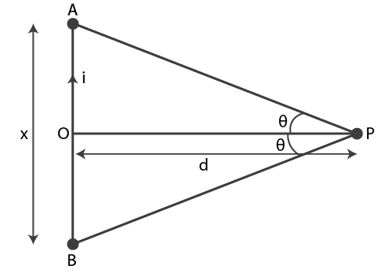The magnetic field on perpendicular bisector:

B = μ0i/4πd [sin θ + sin θ]

= μ0i/4πd [2x/√(x2+4d2)]

If d >>> x, then

B = μ0i/4πd [2x/2d]

B ∝ 1/d2

If d << x, then

B = μ0i/4πd [2x/x]

B ∝ 1/d

Question 15: Consider a 10 cm long piece of a wire which carries a current of 10A. Find the magnitude of the magnetic field due to the piece at a point which makes an equilateral triangle with the ends of the piece.

Solution: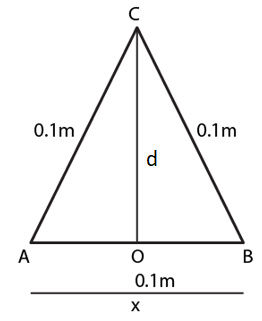Magnetic field at a point on the perpendicular bisector:

B = μ0i/2πd [x/√(x2+4d2)] ….(1)

where, x = length of the wire.

Consider, d is the distance between point C and the midpoint O.

Using Pythagoras theorem,

(BC) 2 = (OB) 2 + (OC) 2

(OC) 2 = (0.1) 2 – (0.05) 2

OC = 0.086 m = d

(1)=>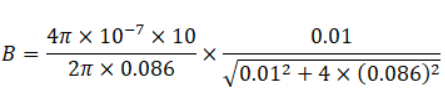B = 2.32 x 10-5 x 0.502 = 1.16 x 10-5 T

Question 16: A long, straight wire carries a current i. Let B1 be the magnetic field at a point P at a distance d from the wire. Consider a section of length ℓ of this wire such that the point P lies on the perpendicular bisector of the section. Let B2 be the magnetic field at this point due to this section only. Find the value of d/ℓ so that B2 differs from B1 by 1%.

Solution:

The magnetic field induced in the wire:

B1 = μ0i/2πd

The magnetic field due to a section of length l on the perpendicular bisector:

B2 = μ0i/4πd x [2l/√(l2+4d2)]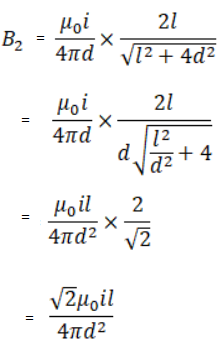Now, B1 > B2

Also, we are given with (B1 – B2)/B1 = 1/100

=> B2 = 0.99 B1

On substituting B1 and B2 in above equation, it turned as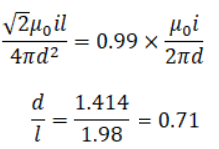Question 17: Figure shows a square loop ABCD with edge length a. The resistance of the wire ABC is r and that of ADC is 2r. Find the magnetic field B at the centre of the loop assuming uniform wires.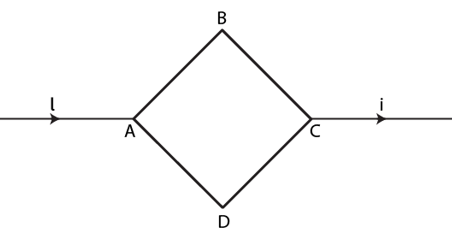Solution: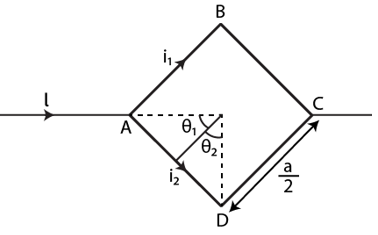Let i1 and i2 currents and r and 2r be the resistances in wires ABC and ADC, i1/i2 = 2/1

i1 – 2i2 = 0 …(1)

i1 + i2 = i ….(2)

Solving (1) and (2), we have

i1 = 2i/3 and i2 = i/3

And, magnetic field at centre O due to wire AB and BC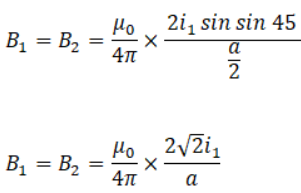Magnetic field at centre o due to wire AD and DC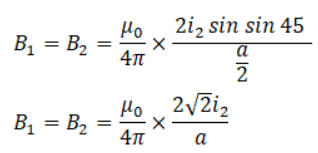Also, i1 = 2i2

and B1 = B2 > B3 = B4

Net magnetic field at centre:

Bnet = B1 + B2 – (B3 + B4)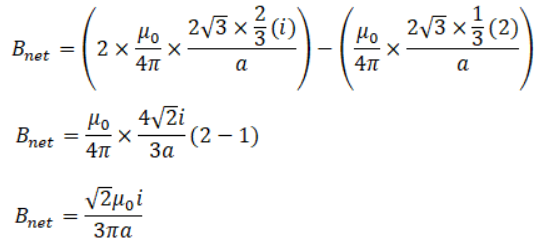Question 18: Figure shows a square loop of edge a made of a uniform wire. A current i enters the loop at the point A and leaves it at the point C. Find the magnetic field at the point P which is not the perpendicular bisector of AB at a distance a/4 from it.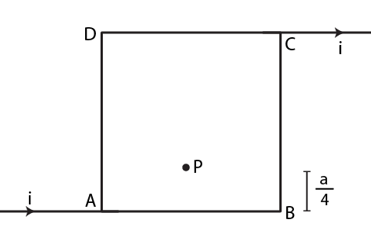Solution: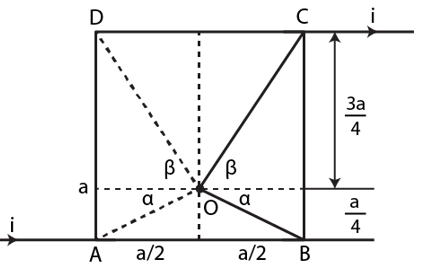Magnetic field due to AB:

BAB = μ0i/4π x i/2(a/4) x [2sin(90- α)] = μ0i/4π x i/2(a/4) x [2 cos α] …(1)

Cos α = (a/2)/AO

From figure,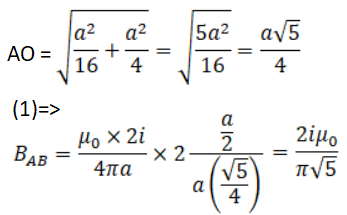Similarly, magnetic field due to DC

BDC = [μ0ix4x2]/[4πx3a] x [cos β] …(2)

From figure,

Cos β = (a/2)/DO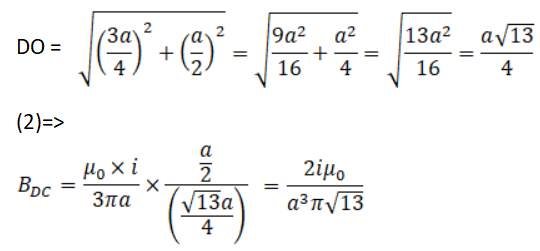The magnetic field due to AD and BC are equal and opposite, so net magnetic field

Bnet = BAB – BDC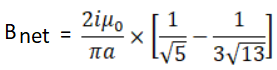Question 19: Consider the situation described in the previous problem. Suppose the current i enters the loop at the point A and leaves it at the point B. Find the magnetic field at the centre of the loop.

Solution:

Magnetic vector B due to BC and due to AD at PT equal in magnitude and opposite in direction. So, net vector B = 0

Similarly, due to AB and CD at P = 0

The net vector B at the centre of the square loop = 0

Question 20: The wire ABC shown in figure forms an equilateral triangle. Find the magnetic field B at the centre O of the triangle assuming the wire to be uniform.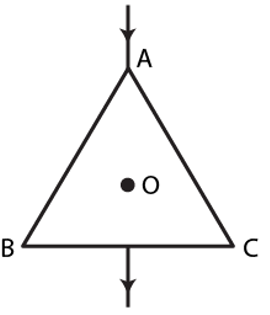Solution: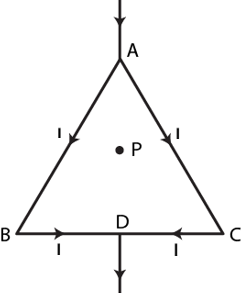Let current 2I flow through the circuit.

Since the wire is uniform, the current is dividing equally between AB and AC arms.

Let “B” be the magnetic field induced at point P due to wire AB and wire AC

and B’ be the magnetic field at P due to wire BD and DC.

So, Net magnetic field at P = B+ B’ – B – B’ = 0

Question 21: A wire of length ℓ is bent in the form of an equilateral triangle and carries an electric current i. (a) Find the magnetic field B at the centre.

(b) If the wire is bent in the form of a square, what would be the value of B at the centre?

Solution: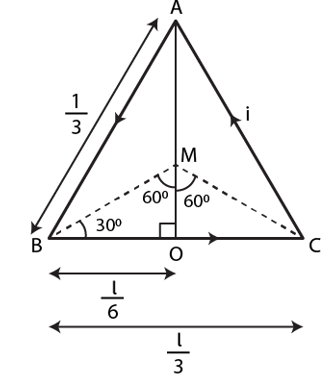Let ABC be the equilateral triangle with each side measure l/3 and angle 60 degrees.

(a) In triangle AOB,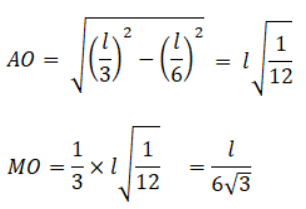Let “d” be the separation of the point from the wire.

d= MO = l/(6√3)

Magnetic field induced due to current in wire BC:

B = μ0i/4πd x 2 sin 60o

=> B = μ0i/4πl x 6√3 x 2√3 = 36μ0i/4πl

Since all the wires are equal, so the net magnetic field at point M due to AB, BC and CA is

Bnet = 3B = 3 x 36μ0i/4πl = 27μ0i/πl

It is perpendicular to the plane outward when the current flows anticlockwise and inward when the current flows clockwise.

(b)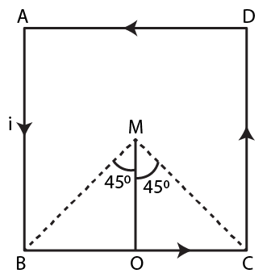The induced magnetic field due to electric current in wire BC:

B = μ0i/4πd x 2 sin 45o

From figure, d = l/8

=>B = [2√2 μ0i]/πl

Given: All the wires are equal, so net magnetic field at point M = 4 × Magnetic field due to wire BC.

=> Bnet = 4 B = [8√2 μ0i]/πl

Question 22: A very long wire carrying a current i is bent to form a plane at an angle α. Find the magnetic field B at a point P on the bisector of this angle situated at a distance x from the vertex A.

Solution: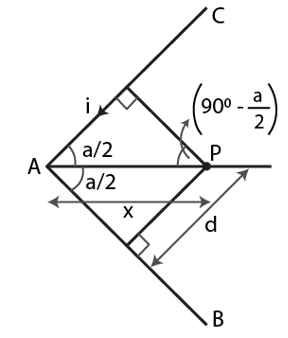From figure, sin(α/2) = d/x

=> d = x sin(α/2)

Where d = separation of the point from the wire.

Let θ1 and θ2 angles made by A and C with P:

θ1 = 90 – α/2 and θ2 = 900

Now, the magnetic field due to current in wire AC: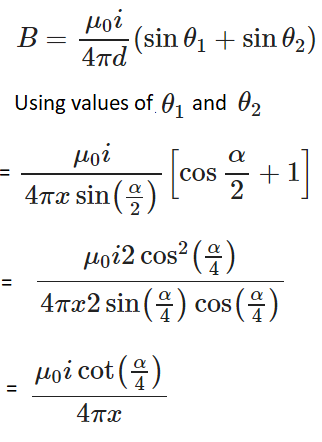The net B due to wires AB and AC: Bnet = 2B = μ0icot(α/2)/2πx

Question 23: Find the magnetic field B at the centre of a rectangular loop of length ℓ and width b, carrying a current i.

Solution: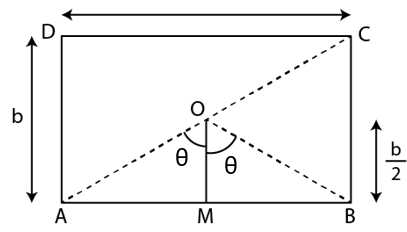Here OM = d = b/2

In triangle, AOM,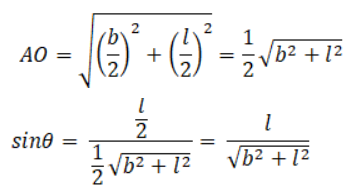Induced magnetic field due to AB:

B = μ0i/4πd x 2 sin θ

B = μ0i/4π(b/2) x 2l/√[l2+b2]

B = μ0i/πb x l/√[l2+b2]

Similarly, the induced magnetic field due to current in BC:

B = μ0i/πl x b/√[l2+b2]

Now,

Magnetic field due to CD = Magnetic field due to current in wire AB = B

Magnetic field due to current in wire DA = Magnetic field due to current in wire BC = B’

Bnet = l(B + B’)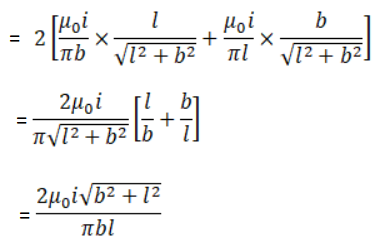Question 24: A polygon of n equal sides is formed by bending a current carrying wire to total length 2πr which carries a current i.

(a) Find the magnetic field B at the center of the n-sided polygon.

(b) By letting n → ∞, derive the expression for the induced magnetic field at the center of a circular current carrying wire.

Solution: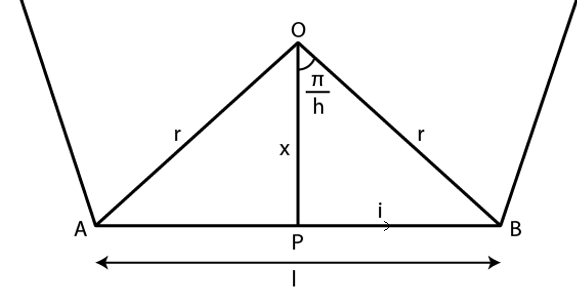(a) For a polygon of n equal sides:

Central angle = 2π/n

Tan θ = l/2x => x = l/(2 Tan θ)

If angle is small, then l/2 = πr/n

Using Biot–Savart’s law for one side of the n-sided polygon,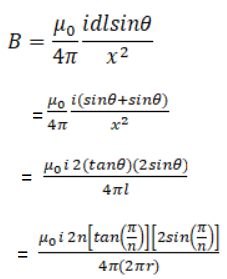[By substituting  given values]

For n-sided polygon:

B’ = nB

(b) When n → ∞, polygon tends to a circle with radius r and magnetic field will tend toward

B = μ0i/2r

Question 25: Each of the batteries shown in figure has an emf 5V. Prove that the magnetic field B at the point P is zero for any set of values of the resistances.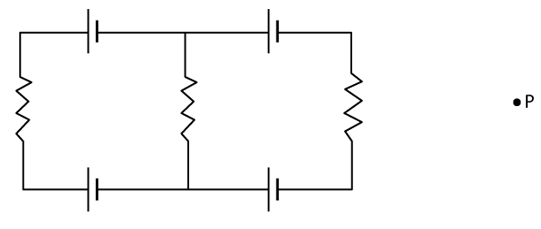Solution:

By applying Kirchhoff’s Voltage Law, net current in the circuit = 0

So, Net magnetic field “A” at the point P = 0

Induced magnetic field at point P is independent of the values of the various resistances in the electric circuit.